# RBSE Maths Class 8 Chapter 14: Important Questions and Solutions

RBSE Maths Chapter 14 – Area Mathematics Class 8 Important questions and solutions are available here. The additional, exercise important questions and solutions of Chapter 14, available at BYJU’S, contain step by step explanations. It can be used as study material for facing the board exams. All these important questions are based on the new pattern prescribed by the RBSE. Students can also get the syllabus and textbooks on RBSE Class 8 solutions.

Chapter 14 of the RBSE Class 8 Maths will help the students to solve problems related to the area of a trapezium, area of a rhombus, area of a quadrilateral, area of a polygon, area of an irregular polygon.

### RBSE Maths Chapter 14: Additional Questions and Solutions

Question 1: State whether the following statements are true/false.

A] Area of a parallelogram, whose base is 6.5 cm and height 4 cm is 26 cm2.

B] 1 meter = 100 square cm.

C] 1 hectare = 10000 square meter.

D] If the diagonals of a rhombus are 24 cm and 7 cm, then the area of the rhombus is 168 cm2.

Solution:

A] True B] True C] True D] False

Question 2: Fill in the blanks.

A] Area of triangle = _____ * base * height.

B] Area of trapezium = [½] * sum of parallel sides * _____.

C] Diagonals of a rhombus are ______ to each other.

D] Area of a rhombus = [½] * _____.

E] Area of rectangle = Length x _____.

Solution:

A] [½] B] height C] perpendicular D] product of diagonals E] breadth

### RBSE Maths Chapter 14: Exercise 14.1 Textbook Important Questions and Solutions

Question 1: The length of two perpendicular sides of a trapezium are 10 cm and 16 cm. The perpendicular distance between them is 8 cm. Find the area of the trapezium.

Solution:

Area of trapezium = [1 / 2] * height * [sum of the parallel sides]

= [1 / 2] * 8 * [10 + 16]

= [1 / 2] * 8 * 26

= [1 / 2] * 208

= 104

The area of the trapezium is 104 square centimeters.

Question 2: The roof of the building is in a particular shape, as shown in the figure below. If all the dimensions are of equal length, then find the area of the whole design.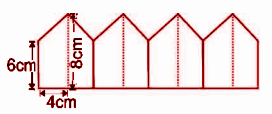Solution:

Every shape in the figure is a trapezium.

Number of shapes = 4

Area of one shape = [1 / 2] * height * [sum of the parallel sides]

= 2 * ([1 / 2] * (6 + 8) * 4)

= 2 * ([½] * 14 * 4]

= 2 * ([½] * 56)

= 2 * 28

= 56 cm2

Area of the entire shape = 4 * Area of one shape = 4 * 56 = 224cm2.

Question 3: The area and height of trapezium are 34cm2 and 4 cm. One of its parallel sides is 10cm. Find the length of another parallel side.

Solution:

Let the length of the required parallel side be ‘x’ cm.

Area of trapezium = [1 / 2] * height * [sum of the parallel sides]

34 = [1 / 2] * 4 * [10 + x]

34 = 2 * [10 + x]

34 = 20 + 2x

34 – 20 = 2x

14 = 2x

14 / 2 = x

7 = x

Hence, the length of another parallel side is 7 cm.

Question 4: The top surface of a platform is In the shape of a regular octagon, as shown in the figure. Find the area of the octagonal surface.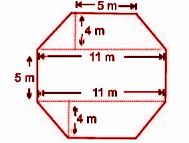Solution:

Required Area = Area of trapezium above + Area of rectangle + Area of lower trapezium

= [1 / 2] * (5 + 11) * 4 + 11 * 5 + [1 / 2] * [11 + 5] * 4

= [1 / 2] * (16) * 4 + 55 + [1 / 2] *  * 4

= 32 + 55 + 32

= 119m2

Question 5: Length between the opposite vertex of the rhombus-shaped plot is 12.5 m and 10.4 m. Find the total cost of making this plot as a flat surface if the cost of making a flat surface per square meter is Rs. 180.

Solution:

Area of rhombus-shaped plot = [1 / 2] * product of diagonals

= [1 / 2] * [12.5 * 10.4]

= [1 / 2] * 130

= 65m2

Cost of making the plane this plot as a flat surface = 65 x Rs. 180 = Rs 11,700.

Question 6: Find the area of the combined rhombus-shaped tiles as given in the figure.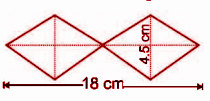Solution:

Area of rhombus-shaped tiles = [1 / 2] * product of diagonals

= 2 * ([1 / 2] * [4.5] * 9)

= 2 * ([1 / 2] * 40.5)

= 2 * [20.25]

= 40.5 cm2

Question 7: The field of the Kalyan is in the form of a quadrilateral. The diagonal of this field is 220 m and, the perpendiculars dropped on it from the remaining opposite vertices are 80 m and 130, respectively. Find the area of the field.

Solution:

Area of quadrilateral shaped form = [1 / 2] * diagonal * [sum of the length of perpendicular on the diagonal]

= [1 / 2] * 220 * [80 + 130]

= [1 / 2] * 220 * 210

= [1 / 2] * 46200

= 23100 m2

Question 8: Fill in the blanks

(i) Area of the rhombus is _____ product of the diagonals.

(ii) Both the diagonals of the scalene quadrilateral is always _____.

(iii) Area of the _____ quadrilateral can be found through the formula height * ([sum of parallel sides] / 2)

(iv) The quadrilateral whose unequal diagonals bisect each other is called _____.

Solution:

(i) half

(ii) unequal

(iii) trapezium

(iv) rhombus

### RBSE Maths Chapter 14: Exercise 14.2 Textbook Important Questions and Solutions

Question 1: Find the area of the polygon according to the measurement given in the figure.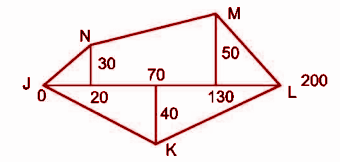Solution: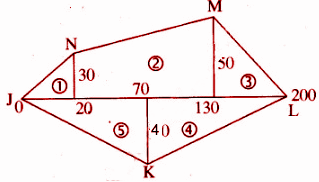Area of polygon JKLMN = Area of figure 1 + Area of figure 2 + Area of figure 3 + Area of figure 4 + Area of figure 5

= [½] * 20 * 30 + [½] * [30 + 50] * [130 – 20] + [½] * [200 – 130] * 50 + [½] * [200 – 70] * 40 + [½] * 70 * 40

= 300 + 4400 + 1750 + 2600 + 1400

= 10450m2

Question 2: Each side of the hexagon ABCDEF has a side of length 5 cm as given in the figure. Riya and Riema find the area of the region by dividing it into two parts into two different manners. Compare the area in both cases.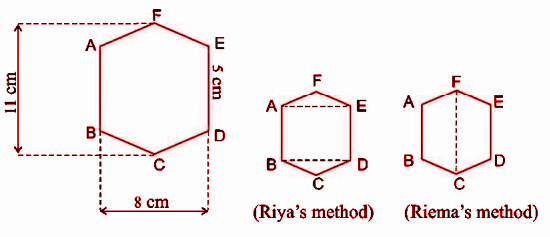Solution:

Ria’s Method:

Area of hexagonal shape ABCDEF = Area of ▵AFE + Area of rectangle AEDB + Area of ▵BCD = [½] * 8 * 3 + 8 * 5 + [½] * 8 * 3

= 12 + 40 + 12

= 64 cm2

Reima’s Method:

Area of hexagonal shape ABCDEF = Area of trapezium ABCF + Area of trapezium FCDE

= [½] * [5 + 11] * 4 + [½] * [5 + 11] * 4

= 32 + 32

= 64cm2

Hence, both areas are the same.

Question 3: The measurement of each part of the farm of Ramlal is given below. Find the total cost of ploughing at the rate of Rs. 4 per meter.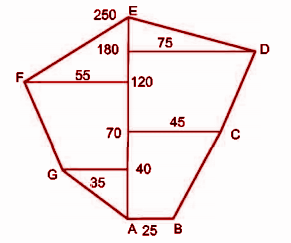Solution: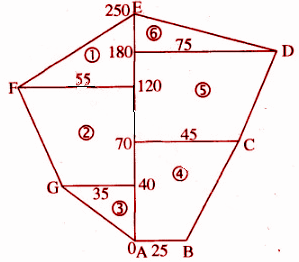Area of field = Area of figure 1 + Area of figure 2 + Area of figure 3 + Area of figure 4 + Area of figure 5 + Area of figure 6

= [½] * 55 * (250 – 120) + [½] * (55 + 35) * (120 – 40] + [½] * 35 * 40 + [½] * (25 + 45) * 70 + [½] * (45 + 75) * (180 – 70) + [½] * 75 * (250 – 180)

= 3575 + 3600 + 700 + 2450 + 6600 + 2625

= 19550m2

Cost of ploughing at the rate of = Rs 4 per meter

Cost of ploughing 19550 sq. units farm = 19550 * 4 = Rs 78,200.

The required amount is Rs. 78200.

### RBSE Maths Chapter 14: Additional Questions and Solutions

Question 1: Azhar has a trapezium-shaped farm. He divides it into three parts as shown in the figure below. Show that the area of the trapezium PQRS = area of triangle SPT + area of rectangle STUR + area of triangle RUQ. Also, compare it with the area of trapezium PQRS.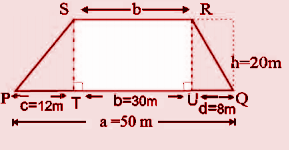Solution:

Area of ▵SPT = [base * height] / 

= [PT * ST] / 

= [12 * 20] / 

= [240 / 2]

= 120m2

Area of rectangle STUR = Length x Breadth = TU * TS = 30 * 20 = 600m2

Area of ▵RUQ = [base * height] /  = [UQ * RU] /  = [8 * 20] /  = [160 / 2] = 80m2

Area of trapezium PQRS = Area of ▵SPT + Area of rectangle STUR + Area of ▵RUQ

= 120 + 600 + 80

= 800m2 ——- (1)

Area of trapezium PQRS = [½] * [a +b] * h

= [½] * [50 + 30] * 20

= 800m2 ——- (2)

From (1) and (2), the area of trapezium PQRS is the same when calculated by both ways.

Question 2: Is trapezium having different parameters in the same area? Prove with the help of the data given in the following figures.Solution:

(i)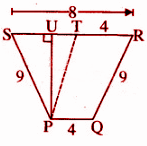Perimeter of trapezium = 4 + 9 + 8 + 9 = 30 units

In the above figure, PT is parallel to QR and PU is perpendicular to RS.

PT || QR and PQ || TR.

Hence, PQRT is a parallelogram.

PT = QR = 9 unit and TR = PQ = 4 units

ST = RS – RT = 8 – 4 = 4 units

Now in ▵PTS, s = [PT + TS + SP] /  = [9 + 4 + 9] /  = 22 / 2 = 11 units

Area of ▵PTS = √s (s – a) (s – b) (s – c)

= √11 [11 – 9] * [11 – 4] * [11 – 9]

= √11 [2 * 7 * 2]

= √308

= 2 √77

= 17.54 square units

Area of ▵PTS = [½] * ST * PU

17.54 = [½] *  * {PU}

17.54 = 2PU

PU = [17.54] /  = 8.77

Area of trapezium PQRS = [½] * [PQ + RS] * PU

= [½] * [4 + 8] * 8.77

= 52.62 square units

(ii) Perimeter of trapezium = 7 + 6 + 6 + 10 = 29 units

Area of trapezium = [½] * [height] * [sum of parallel sides]

= [½] * 6 * [10 + 6]

= 48 square units

(iii)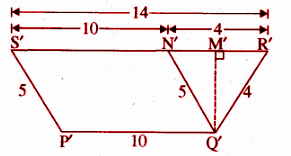Perimeter of trapezium P’Q’R’S’ = 10 + 4 + 14 + 5 = 33 units

Q’N’ II S’P’ and Q’M’ ⟂ S’R’

Q’N’ II S’P’ and P’Q’ || S’R’ implies that P’Q’ II S’N’

P’Q’N’S’ is a parallelogram.

Q’N’ = P’S’ = 5 units and S’N’ = P’Q’ = 10 unit

R’N’= S’R’ – S’N’ = 14 – 10 = 4 unit

In triangle Q’R’N’, a = 4, b = 5, c = 4

s = [a + b + c] /  = [4 + 5 + 4] /  = 13 / 2 units

Area of triangle Q’R’N’ = √s (s – a) (s – b) (s – c) = 7.80 square units

Area of triangle Q’R’N’ = [½] * N’R’ * Q’M’

7.80 = [½] *  * [Q’M’]

7.80 * 2 = 4 Q’M’

Q’M’ = 3.9 units

Area of trapezium P’Q’R’S’ = [½] * [P’Q’ + R’S’] * Q’M’

= [½] * [10 + 14] * 3.9

= 12 * 3.9

= 46.8 square units

From (i), (ii) and (iii), it is clear that trapezium with different perimeters cannot have the same area.

Question 3: Match the following.

 Question Answer choices 1] Area of trapezium a] [½] * product of the diagonals 2] Area of the rhombus b] [½] * height * [sum of parallel sides] 3] Side of a square whose area is 1 hectare. c] 20 meter 4] Length of the rectangle whose breadth is 5 meter and the area is 1m2. d] 100 meter

Solution:

1] – b]

2] – a]

3] – d]

4] – c]

Question 4: In a parallelogram ABCD, AB = 35 cm and height is 12 cm corresponding to the side BC = 25 cm. Find the corresponding height.

Solution:

Area of a parallelogram ABCD = 35 * 12 = 25 * height corresponding to BC

Height corresponding to side BC

= [35 * 12] / 

=  / 

= 16.8cm

Question 5: In the given figure BC = 30cm. Find the area of triangle ABC.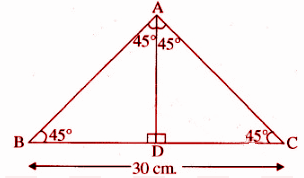Solution:

In triangle ABD,

∠DAC = ∠DCA

From (1) and (2)

AD = [½] * BC = [½] * 30 = 15cm

Area of triangle ABC = [½] * base * height

= [½] * BC * AD

= [½] * 30 * 15

= [½] * 450

= 225cm2

Question 6: In the given figure, ABCD is a trapezium-shaped field. AB || DC, DC = 25 cm, CE = 12 cm. and EB = 10 cm. Find the area of the quadrilateral.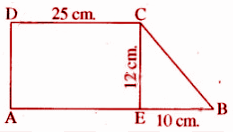Solution:

Area of trapezium ABCD = Area of rectangle AECD + Area of right angle triangle CEB

= 25 * 12 + [10 * 12] / 

= 300 + 60

= 360 cm2

Question 7: The perimeter of a rhombus is 52 cm. Distance between two parallel sides is 12 cm. Find the area of rhombus.

Solution:

Perimeter of rhombus = 52 cm.

One side of rhombus = 52 / 4 = 13 cm

Height of the rhombus = 12 cm

Area of rhombus = base * height = 13 * 12 = 156 cm2

Question 8: Area of the trapezium is 140 cm2 and height is 10cm. The difference between parallel sides is 4 cm. Find the lengths of both parallel sides.

Solution:

Let a and b be the lengths of parallel sides where a > b.

Area of trapezium = [½] * (sum of parallel sides) * height

140 = [½] * [a + b] * 10

140 * 2 = (a + b) * 10

280 = (a + b) * 10

a + b = [280 / 10] = 28

a + b = 28 —- (1)

a – b = 4 ——- (2)

Solving the above two equations,

2a = 32

a = 32 / 2

a = 16 cm

Put a = 16 in (1)

16 + b = 28

b = 28 – 16

b = 12 cm

Hence, the required lengths of parallel sides are 16 cm and 12 cm, respectively.

Question 9: In a given figure ABCD is a trapezium in which AB || DC and DA ⟂ AB. If AB = 13 cm, AD = 8 cm and CD = 7 cm, then find the area of trapezium.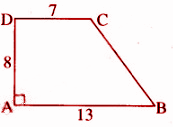Solution:

Area of trapezium = [½] * (sum of parallel sides) * height

= [½] * [7 + 13] * 8

= [½] *  * 

= 80 square cm.

Question 10: Polygon ABCDE is divided in different parts as shown in figure. If AD = 8 cm, AH = 6 cm, AG = 4 cm, AF = 3 cm and BF = 2 cm, CH = 3cm, EG = 2.5 cm. Then find the area of the polygon.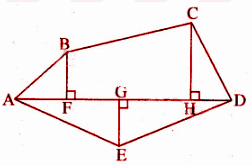Solution:

Area of polygon ABCDE = Area of right ▵AFB + Area of trapezium BFHC + Area of right ▵CHD + Area of right ▵EGD + Area of right ▵AGE

= [½] * AF * BF + [½] * [BF + CH] * FH + [½] * HD * CH + [½] * GD * GE + [½] * AG * GE

= [½] * 3 * 2 + [½] * [2 + 3] * [6 – 3] + [½] * [8 – 6] * 3 + [½] * [8 – 4] * 2.5 + [½] * 4 * 2.5

= 3 + 7.5 + 3 + 5 + 5

= 23.5cm2

Question 11: Find the area of polygon MNOPQR if MR = 9 cm, MD = 7 cm, MC = 6 cm, MB = 4cm, MA= 2 cm, NA,OC, QD and RB are perpendiculars on diagonal MP.

Solution:

Area of polygon MNOPQR = Area of ▵MAN + Area of trapezium ACON + Area of ▵OCP + Area of ▵QDP + Area of trapezium DBRQ + Area of ▵RBM

= [½] * AM * NA + [½] * [NA + OC] * AC + [½] * CP * OC + [½] * DP * QD + [½] * [BR + DQ] * BD + [½] * MB * BR

= [½] * 2 * 2.5 + [½] * [2.5 + 3] * 4 + [½] * 3 * 3 + [½] * 2 * 2 + [½] * [2.5 + 2] * 3 + [½] * 4 * 2.5

= 2.5 + 11 + 4.5 + 2 + 6.75 + 5

= 31.75 cm2

Question 12: The transverse cut of a canal is trapezium-shaped. The canal is 10 meters wide at the top and 6 meters wide at the bottom. The area of the transverse cut is 72 m2, then find its depth.

Solution:

Area of trapezium = [½] * (sum of parallel sides) * height

72 = [½] * (10 + 6) * height

72 = 8 * height

Height = 72 / 8

Height = 9 meters

Hence, the depth is 9 meters.

Question 13: The diagonals of a rhombus are 15 cm and 36 cm. Find its perimeter and area.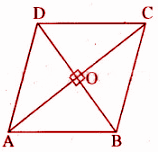Solution:

Area of rhombus = [½] * product of the diagonals = [½] * 15 * 36 = 270 cm2

We know that the diagonals of a rhombus bisect each other at a right angle.

OA = OC = [½] AC = [½] * 36 = 18cm and OB = OD = [½] * MD = [½] * 15 = 7.5 cm.

AB = √OA2 + OB2

= √182 + (7.5)2

= √324 + 56.25

= √380.25

= 19.5 cm

Perimeter of rhombus = 4 * AB = 4 * 19.5 = 78 cm.

Question 14: As shown in the picture, a farm is similar in the shape of polygon ABCDEF. All the measurements are given in meters. Find the area of polygon ABCDEF.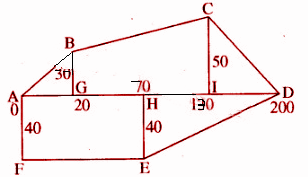Solution: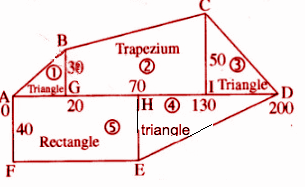Area of polygon ABCDEF = Area of figure 1 + Area of figure 2 + Area of figure 3 + Area of figure 4 + Area of figure 5

= [½] * 20 * 30 + [½] * [30 + 50] * [130 – 20] + [½] * [200 – 130] * 50 + [½] * [200 – 70] * 40 + 70 * 40

= 300 + 4400 + 1750 + 2600 + 2800

= 11850 square meter

Question 15: Find the area of the field shown below. All the measurements are in meters.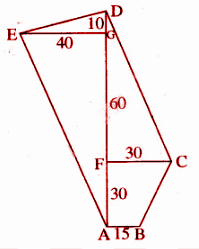Solution:

Area of polygon ABCDEA = Area of ▵ADE + Area of ▵DFC + Area of trapezium ABCF

= [½] * AD * EG + [½] * DF * FC + [½] * [AB + FC] * AF

= [½] * 90 * 40 + [½] * 60 * 30 + [½] * [15 + 30] * 30

= 1800 + 900 + 675

= 3375 meters

Question 16: Area of a rhombus ABCD is 264 cm2. If the length of its one diagonal AC = 24 cm, then find the length of diagonal BD.

Solution:

Area of rhombus = [½] * product of diagonals

Area of rhombus = 264 cm2

Diagonal AC = 24 cm

Diagonal BD = ?

264 = [½] * 24 * x

[264 * 2] / 24 = x

22 = x

Diagonal BD = 22 cm

Question 17: The area of a rhombus is 360cm2. If the length of one of its diagonals is 20 cm, then find the length of another diagonal.

Solution:

Area of rhombus = [½] * product of diagonals

360 = [½] * 20 * x

360 * 2 = 20 * x

720 = 20x

720 / 20 = x

36 = x

The length of the other diagonal is 36cm.

Question 18: Find the area of the given figure. All the measurements are in meters.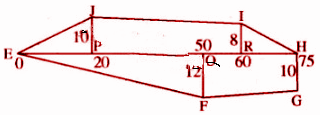Solution:

Total area of figure = Area of ▵PEJ + area of trapezium PRIJ + area of ▵IRH + area of trapezium HGFQ + area of ▵EQF

Area of ▵PEJ = [½] * Base * Height

= [½] * 10 * 20

= 100 m2

Area of trapezium JPRI = [½] * (sum of parallel sides) * (distance between parallel sides)

= [½] * (10 + 8) * (60 – 20)

= [½] * 18 * 40

= 360 m2

Area of ▵IRH = [½] * base * height

= [½] * 8 * (75 – 60)

= 60 m2

Area of trapezium FGHQ = [½] * (sum of parallel sides) x (distance between parallel sides)

= [½ ] * (10 + 12) * (75 – 50)

= [½] * 22 * 25

= 275 m2

Area of ▵EQF = [½] * base * height

= [½ ] * 12 * 50

= 300 m2

Total area of figure = (100 + 360 + 60 + 275 + 300) = 1095 square meters.